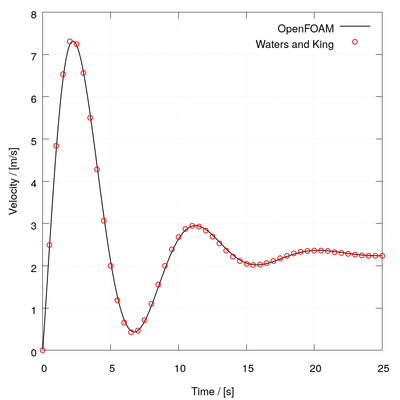## Results🔗

The predictions are compared against the analytical solution for velocity given by Waters and King 

$u(y,t) = \frac{3}{2}(1 - y^2) + \sum\limits_{k=1}^\infty A_k(t)B_k(t),$ $A_k(t) = e^{-b_k t} \begin{cases} \frac{b_k-a_k^2}{c_k}\sinh c_k t + \cosh c_k t, & \text{if } b_k \geq a_k, \\ \frac{b_k-a_k^2}{c_k}\sin c_k t + \cos c_k t, & \text{if } b_k \lt a_k, \end{cases}$ $B_k(y) = \frac{48(-1)^k}{(2k -1)^3\pi^3} \cos \frac{2k - 1}{2}\pi y,$

where

$a_k = \frac{2k - 1}{2}\pi \sqrt{E},\; b_k = \frac{1+\beta a_k^2}{2},\; c_k = \sqrt{|b_k^2 - a_k^2|}.$